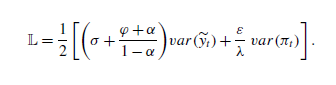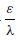# Inflation and output gap are different order of magnitude

Hello, everyone, I am working on a paper about macroprudential and monetary policy, I need find the optimal parameters in moneraty policy equation , which is according to welfare loss function L = alpha1 * var(pie) + alpha2 * var (y). But when I give a monetary policu shock . the variance of inflation is 4.919139096552384e-08 , the variance of output is 1.27575642287463e-06, they are not in same order of magnitude, I wonder how to solve is, thank you!Hi, I am not sure I understand how the variances implied by a monetary policy shock could be of interest… But I suppose that the relative variances should depend on the (relative) values you have chosen for \alpha_1 and \alpha_2. So the fact that the output is more volatile is not necessarily surprising.

Best,
Stéphane.

Thank you, Stéphane. what you means if welfare loss function like in Jordi Galı´ - Monetary Policy,Inflation,and the Business Cycle 2008 ( chapter 4 )which can be used in my model by the way* 100 , if I am not misunderstand,because my code is nonlinear?

Best wishes,
wu_Justin.

You need to be more precise on what your are tying to do, where the problem is and what the objects you are talking about are.
In the loss function you describe the \frac{\varepsilon}{\lambda} term is nonlinear in the parameters, but not in the variables. Regardless, if your steady state is efficient, then a first order approximation is sufficient. See https://github.com/JohannesPfeifer/DSGE_mod/tree/master/Gali_2015
If your steady state is distorted, you need a nonlinear model with a second order approximation. See e.g. https://github.com/JohannesPfeifer/DSGE_mod/tree/master/Born_Pfeifer_2018/Welfare

Thank you, professor. I wii have a think about it.